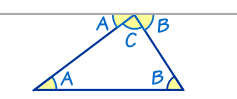# Triangles Contain 180°### In a triangle, the three interior angles always add to 180°:

A + B + C = 180°

Try it yourself (drag the points):

We can use that fact to find a missing angle in a triangle:

### Example: Find the Missing Angle "C"Fill in what we know:38° + 85° + C = 180°
Rearrange C = 180° − 38° − 85°
Calculate:C = 57°

## Proof

This is a proof that the angles in a triangle equal 180°:The top line (that touches the top of the triangle) is
running parallel to the base of the triangle.

So:

• angles A are the same
• angles B are the same

And you can easily see that A + C + B does a complete rotation from one side of the straight line to the other, or 180°